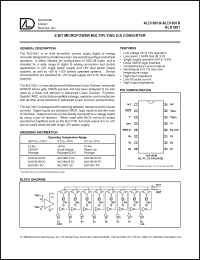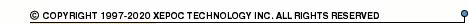More than478 726 registered clientsALD1801 series datasheets. Manufacturer: Advanced Linear Devices, Inc..

 ALD1801ADC Micropower multiplying D/A converter in 16-pin CERDIP package. Operational temperature range from -55°C to 125°C. Datasheet*) ALD1801APC Micropower multiplying D/A converter in 16-pin PDIP package. Operational temperature range from 0°C to 70°C. Datasheet*) ALD1801ASC Micropower multiplying D/A converter in 16-pin SOIC package. Operational temperature range from 0°C to 70°C. Datasheet*) ALD1801BDC Micropower multiplying D/A converter in 16-pin CERDIP package. Operational temperature range from -55°C to 125°C. Datasheet*) ALD1801BPC Micropower multiplying D/A converter in 16-pin PDIP package. Operational temperature range from 0°C to 70°C. Datasheet*) ALD1801BSC Micropower multiplying D/A converter in 16-pin SOIC package. Operational temperature range from 0°C to 70°C. Datasheet*) ALD1801DC Micropower multiplying D/A converter in 16-pin CERDIP package. Operational temperature range from -55°C to 125°C. Datasheet*) ALD1801PC Micropower multiplying D/A converter in 16-pin PDIP package. Operational temperature range from 0°C to 70°C. Datasheet*) ALD1801SC Micropower multiplying D/A converter in 16-pin SOIC package. Operational temperature range from 0°C to 70°C. Datasheet*) ALD1801 8 BIT MICROPOWER MULTIPLYING D/A CONVERTER Datasheet*) ALD1801A 8 BIT MICROPOWER MULTIPLYING D/A CONVERTER Datasheet*) ALD1801B 8 BIT MICROPOWER MULTIPLYING D/A CONVERTER Datasheet*)